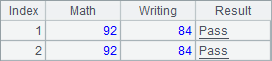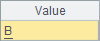• 集算器
教程
函数参考
习题
代码参考
用户参考
外部库使用指南
数据文件工具用户参考
DQL教程
集群管理器使用说明
SPL命令执行器用户参考
• 润乾报表
教程
填报教程
分析教程
报表中心教程
用户参考
程序员参考
高级指南
通用查询
DQL部署集成手册
• 易明建模
用户参考
json参数说明
• 润乾官网

# 多层次参数

## 函数参数的分隔符

 A B 1 Math 92 2 Writing 84 3 =if(B1>=80 && B2>=60,"Pass","Fail") 4 =create(Math,Writing,Result) 5 >A4.insert(0,B1,B2,A3)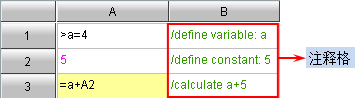A B 1 Math 92 2 Writing 84 3 =if(B1+B2>=180:"A",between(B1+B2,150:180):"B", B1+B2>=120:"C",B1+B2<120:"D") 4 =create(Math,Writing,Rank) 5 >A4.insert(0,A3:Rank,B1:Math,B2:Writing)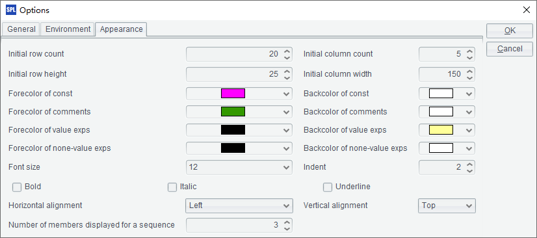A 1 =demo.query("select * from CITIES") 2 =A1.sort(STATEID,NAME) 3 =A1.sort(STATEID,-NAME)

A2A3中均对各个城市的记录做了排序，且均为先按州代码排，同一州的城市再按名称排序。不同的是，A3中的函数在名称前添加了负号，表示按名称排序时，从大到小降序。A2A3中获得的结果如下所示：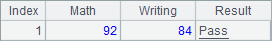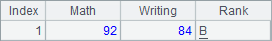A 1 =demo.query("select * from CITIES") 2 =A1.groups(STATEID; sum(POPULATION):Population) 3 =A2.top(-5;Population)

A2中的groups()函数中，分号前的参数用来分组，分号后的参数用来计算汇总值；汇总值的参数，还用到了冒号分隔符，用来定义汇总字段的名称。A2中结果如下：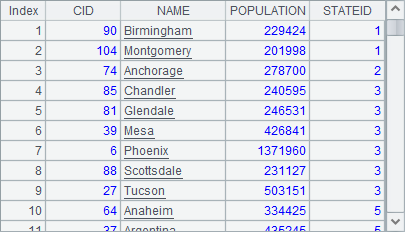A3中的top()函数中，在分号前面定义了取前几位的记录，结果如下：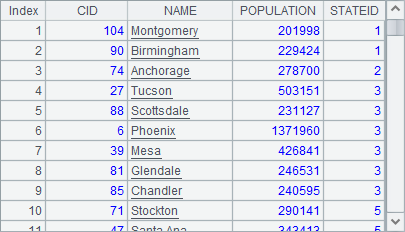A 1 =demo.query("select * from STATES") 2 =demo.query("select EID, NAME, STATE, DEPT, SALARY from EMPLOYEE") 3 =A2.groups(DEPT;count(~):Count) 4 =A2.switch(STATE,A1:NAME; DEPT,A3:DEPT)

A4T.switch()函数中，将不同的字段变换为其它序表中的记录，中间用分号分隔。在A4中，同时使用了逗号、冒号和分号作为函数参数的分隔符，这样的书写方式使得函数的参数层次明晰。A4中的计算结果如下：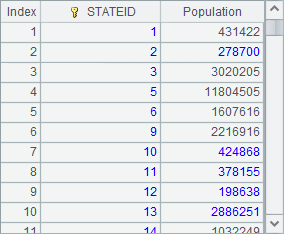## 函数参数的省略

 A B 1 Math 92 2 Writing 84 3 =if(B1>=80 && B2>=60,"Pass","Fail") 4 =create(Math,Writing,Result) 5 >A4.insert(0,A3:Result,B1:Math,B2:Writing) 6 >A4.insert(0,B1,B2,A3)

A5中，冒号后面的参数用来指定插入记录时，某个值对应的字段名。在A6中，使用默认的字段名依次设入字段值，此时可以省略指定字段名的部分。A5A6插入两条记录后，A4中的结果如下：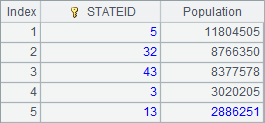A B 1 Math 92 2 Writing 84 3 =if( B1+B2>=180:"A",between(B1+B2,150:180):"B", between(B1+B2,120:150):"C", B1+B2<120:"D")

A3中结果如下：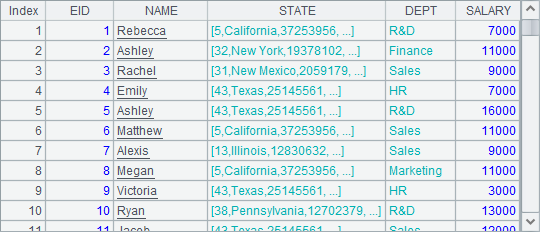A 1 [a,b,c,d,e,f,g] 2 =A1.to(4,) 3 =A1.to(,4)

A2中的表达式相当于=A1.to(4,A1.len())A3中的表达式相当于=A1.to(1,4)。我们可以对比一下A2A3中的结果：